## why remove works different on diff(y(x),x) vs. one...

When doing

```item:=x;
res:=remove(has,item,x);
```

Maple returns, as expected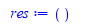But when item is not a single symbol, say sin(x) or diff(y(x),x), then it no longer returns ()

```item:=diff(y(x),x);
res:=remove(has,item,diff(y(x),x));
```

It does not return () but it now returns the input itself, as if it was not removed.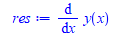Why is that, and how to make it return () also on composite expression?  I tried flatten and inplace option. Otherwise, I will have to do a special test before.

The strange thing is that it works on

```item:=2*diff(y(x),x);
res:=remove(has,item,diff(y(x),x));
```

Now it returns as expected. So now it did remove diff(y(x),x). But it does not remove it when it is on its own. I assume this is by design. But it is not intuitive to the user. One would expect it to work same way on removing `x` or `sin(x)` or `diff(y(x),x)`

## Is it possible to export routines from a personal ...

Is there a way to export routines from a package. The reason I ask, I was answering a question here and I needed two routines I have, that I put together a couple of years ago in a package. I used showstat copied, pasted and edited. Worked, but not great and would utterly fail with anything complicated. e.g. In the two routines pasted  below SignedArea on line 3 c  is c    that caused a minor problem.## Can maple solve an ode using asymptotic expansions...

Can Maple solve an ode by asymptotic expansions methods? series methods (power series or Frobenius series) work only on ordinary expansion point or removable singularity point. For non removable singularity (essential), Maple's dsolve with series method can't solve these. Also, if the RHS of the ode is not analytic at the expansion point, series method do not work.

The asympt command only applies to algebraic expressions.

Does Maple have something similar to Mathematica's AsymptoticDSolveValue  ?

Here is an example. This is an ode with RHS not analytic (has no Taylor series) at x=0

```ode:=diff(y(x),x)+y(x)=1/x;
dsolve(ode,y(x),'series',x=0)```

No solution.  While in Mathematica

```ode = y'[x] + y[x] == 1/x
AsymptoticDSolveValue[ode, y[x], {x, 0, 6}]```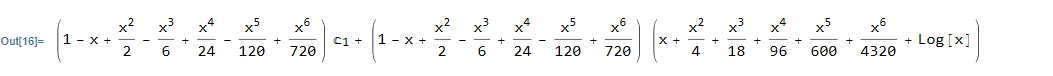Here is another example of ode where the coefficient of y(x) is not analytic at x=0

```ode:=diff(y(x),x)+sqrt(x)*y(x)=0;
dsolve(ode,y(x),'series',x=0)```

No solution. In Mathematica

```ode = y'[x] + Sqrt[x] y[x] == 0
AsymptoticDSolveValue[ode, y[x], {x, 0, 6}]```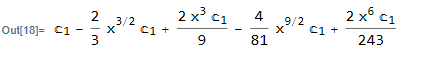Does there exist a package in Maple that can do this? Will this functionality be added in Maple in the future if currently there is no support? ( I searched and could not find anything so far).

One possible workaround I found is to solve the ODE using normal methods (non-series), then apply the asympt command on the solution. But one needs to remove the constant of integration first, Here is the above example done this way

```ode:=diff(y(x),x)+sqrt(x)*y(x)=0;
sol:=dsolve(ode);
Order:=8;
sol:=eval(sol,_C1=1);
asympt(eval(rhs(sol),x=1/y),y);
eval(%,y=1/x)

```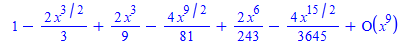Mutliplying the above back by the constant _C1 gives same answer as Mathematica's.

Is the above how one is supposed to do it in Maple? It worked on the above simple example, but need to see if it will work for other examples. it will be better if this was more directly supported. i.e. if asympt will work directly on ode's (or a new command or a new option to dsolve similar to 'series' but call 'asympt').

## provide way to override variable and method in bas...

Maple Object model is somewhat limited.  One of the main reasons to use OOP is to be able to extend base class, and override methods in base class by new methods if needed.

This is described in  https://en.wikipedia.org/wiki/Method_overriding

Method overriding, in object-oriented programming, is a language feature that allows a subclass or child class to provide a specific implementation of a method that is already provided by one of its superclasses or parent classes. It allows for a specific type of polymorphism (subtyping). The implementation in the subclass overrides (replaces) the implementation in the superclass by providing a method that has same name, same parameters or signature, and same return type as the method in the parent class. The version of a method that is executed will be determined by the object that is used to invoke it. If an object of a parent class is used to invoke the method, then the version in the parent class will be executed, but if an object of the subclass is used to invoke the method, then the version in the child class will be executed. Some languages allow a programmer to prevent a method from being overridden.Maple does not allow the extending class to override variables or methods in the base class. Even if the type of the variable or the sigature of the proc is different as long as the name is the same.

This makes it hard to override base class implementation and to do type extension.

Here is a toy example

```restart;

animal:=module()
option object;
export data1;

export move::static:=proc(_self,\$)
print("In Animal class. moving ....");
end proc;
end module;

#create class/module which extends the above
dog:=module()
option object(animal);
export move::static:=proc(_self,\$)
print("In dog class. moving ....");
end proc;
end module;

```

Error, (in dog) export `move` is declared more than onc

All computer languages that supports OOP that I know about supports overriding, The above web page lists some.

A workaround, is if such name conflict occurs, is to come up with new name. So the above example becomes

```
dog:=module()
option object(animal);
export move_the_dog::static:=proc(_self,\$)
print("In dog class. moving ....");
end proc;
end module;
```

And now Maple does not complain. But the whole point of type extension is to override the base class implementation, and not add a new implementation while keeping the base class one there.

Is there a way to do this in Maple? if not in current version, are there plans to add this to future Maple versions?

## Object does not have a moduleIterator error ...

This is another one of those problems that shows up only when I put all my modules in a lib and run the program, and unable to make a MWE in the worksheet.

Here is description of the problem. There is a module which is an object, which has no method. Just variables.

```export SUM_type:=module()
option object;

export starting_index::integer;
export body;
export power_on_x;
export actual_sum;
end module;```

some where inside the program, a list is created of such objects. This problem only shows up when there is ONE object in the list. Where there is 2 or more objects in the list, no problem. The problem shows up here

```for tmp in op(THE_SUMS) do    #BUG IN MAPLE
new_ode := new_ode + tmp:-actual_sum;
od; ```

The above code is from inside a proc in a module. The variable THE_SUMS is the list and tmp is just a local variable.

In the debugger, once I step in the loop, it generates an exception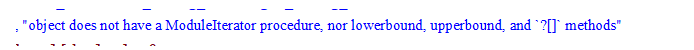The strange thing, this only happens when there is ONE object in the list and not more. Here are screen shot from the debugger window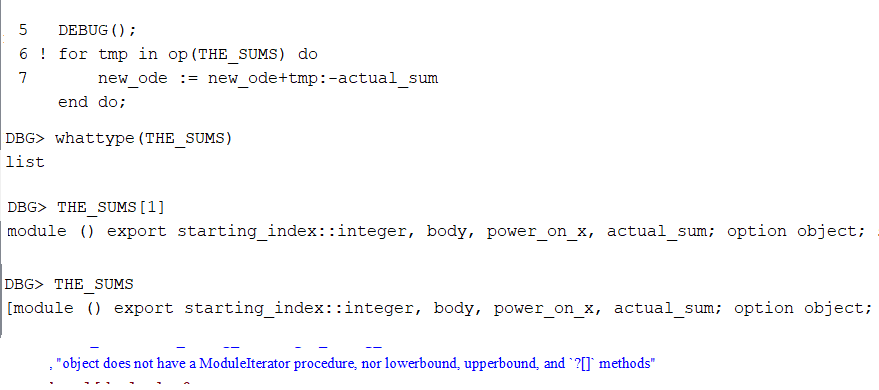To avoid this, I changed the loop to the following, and not it works with one or more entries in the list

```   for N from 1 to nops(THE_SUMS) do
tmp := THE_SUMS[N];
new_ode := new_ode + tmp:-actual_sum;
od;```

And this work. I spend a lot of time trying to make a MWE, but it works ok in worksheet. It looks like a scoping issue. I do not use ModuleIterator, and do not need it. When I added one to the object above just to see what happens, I saw the ModuleItrator being called. I have no idea why Maple wants to call it when there is one object in the list.

Here is one attempt on a MWE. but this gives no error.

```SUM_type:=module()
option object;

export starting_index::integer;
end module;
o:=Object(SUM_type):
o:-starting_index:=1;
L:=[o]:
for item in L do  #OK here since in global name space?
print(item);
od;
```

I am just repoting this in case someone have seen it before. I have a workaround it as I showed, which is to avoid using `for temp in list` when the list has objects in it.

Maple 2021.1 on windows 10

## How to convert a netlist to a ladder schematic?...

Hi Everyone,

I would like to convert a netlist to a ladder schematic by using Draw (Syrup). So far I have tried combinations of &+ and &// and have not achieved the correct result. Please help.

capnet:= "
V  1 0 1
L  1 2 1
C1 2 3 2uF
C2 2 4 3uF
C3 4 3 1uF
C4 4 5 1.5uF
C5 3 5 2.5uF
C6 3 0 3.5uF
C7 5 0 0.5uF
.end":

ckt:= [ V(1), L(1), C1(2.8E-6),
C2(3.E-6),  C3(1.E-6),
C4(1.5E-6), C5(2.5E-6) &+ C6(3.5E-6)
]:
Draw(ckt);

Thanks.

SyrupQuestion.mw

## Question on solve technique...

In the attached, I had to find the required coefficient. Now this has a relatively straight forward set of equation (which apparently form a closed group), so it was easy enough tee see what to do. In a more complicated situation that may be very difficult to see. I was a wondering is there is a better approach to use here?Prove/show the coefficient ofinis(1)(2)(3)(4)(5)(6)(7)(8)(9)(10)(11)## Is the int option continuous backward ?...

The help page says that an option for int is continuous=truefalse. The help page reads

"Specifies whether int looks for discontinuities. " I interpret that as continuous=true means look for discontinuities

and false means do not look. A simple example, however, suggests that it is the opposite.

int(3/(5-4*cos(x)),x=0..2*Pi,continuous=true)=0

int(3/(5-4*cos(x)),x=0..2*Pi,continuous=false)=2*Pi.

I interpret this as continuous=true means "I guarantee the result is continuous, so do not look"

This would be a logical opposite to discont=true in the plot command: discont=true -> continuous=false!

## what are known limitation for running code in head...

I just spend 2 hrs trying to make this work in headless worksheet, but it does not work. I have A worksheet, which calls B worksheet. The call all go through OK and I set it all correctly. Set up the section in worksheet B and document property, etc.. as described in help.

So the call works OK and arguments are passed correctly from A to B.

The problem is that in worksheet B, I wanted to generate a plot in order to save it to postscript file.  This did not work. Nothing gets exported, even  though the plot is actually generated correctly in B.  How do I know this? Becaue B returns the plot back to A, and I can see the plot was correctly generated.  The file .ps never gets created. I look at the folder these worksheets are in, and there is no .ps file created.

But it was not saved to .ps file.  The same code works ok in standard worksheet and plot is saved correctly to .ps file using same code.

I also could not export the plot to JPEG file from worksheet B.  Only thing that worked is exporting some text to .TXT file from B.

Any one knows of limitation to calling one worksheet from another?  It seems somethings work in headless worksheet and some things do not. Which does not make it useful.

You might ask, why Am I doing this? Because I wanted to call that B worksheet later on (if it worked) from a script to do the plot exporting, as the quality is better when in a "worksheet" vs. command line).

But since this does not even work when calling the worksheet from another worksheet, then there is no point of trying it from the command line.

Attached A and B. To try it, please save both worksheets in same folder and simply open A.mw and run it. It will call B.mw

This is the first time I used documentTools package. I did not even know one can call one worksheet from another before.

I suspect it had to do with print(); call becoming inactive in headless worksheet, which causes nothing to be send to the postscript file. But I am not sure. Without print(); one can't export the plot.

I do not understand why is it so hard in Maple to export a plot to a PDF. Why is there not a simple export command to export a plot to PDF like with all the other software out there in the world?  This is the year 2021, not 1980. How many decades does Maplesoft needs to implement export to PDF?

This is on windows 10. Maple 2021.1

This is A.mw

 > restart;
 > currentdir("C:\\tmp\\TEST_MAPLE"); p:=DocumentTools[RunWorksheet]( "B.mw" , [the_function=sin(x),the_variable=x]  ): print("Back from calling worksheet B. Here is the result"); p;>

This is B.mw

Inputs

 > the_function:=0;
 > the_variable:=0;
 > p:=plot(the_function,the_variable=-2*Pi..2*Pi): full_file_name:=cat("C:\\tmp\\TEST_MAPLE\\tmp.ps"); plotsetup(ps,plotoutput=full_file_name): print(p); plotsetup(default): return p;

## How to use smartview option in Plot?...

in my program, I need to generate plot of solution. I only know where the initial condition x value is. So I give to plot the x range as some value around that initial condition location. But this is not perfect, since the command line script will fail if Y value happend to be too large somewhere in this range due to singularity. (This fails only in the command line print driver, not in the worksheet GUI).

I can ofcource limit the y range also, using view=[....] but I do not know how to pick best Y range  automatically in the program to show what is interesting in the plot without doing lots of analysis on the expression.

There is something called smartview which is supposed to be active by default. But it does not seem to be working too well.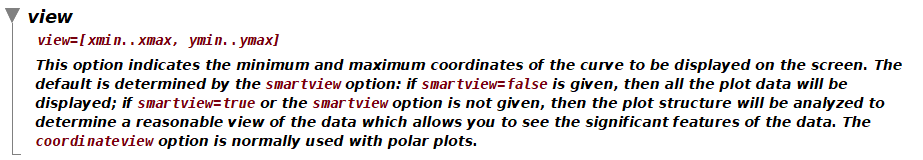Here is an example. This function blows up at value near x=Pi  due to singularity. and I want the plot to automatically limit the Y range without having to specify manually the y view.

```restart;
sol:=exp(-3^(1/2)*(cos(x)-1)/sin(x)):
plot(sol,x=0..2*Pi);
```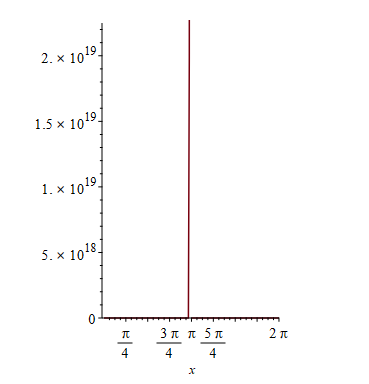So clearly "smartview" did not do it or I am not using it correctly. But it is supposed to be "active"?

Compare the same thing with Mathematica Plot where this  is handled automatically by Plot

```sol = Exp[-3^(1/2)*(Cos[x] - 1)/Sin[x]];
Plot[sol, {x, 0, 2*Pi}]```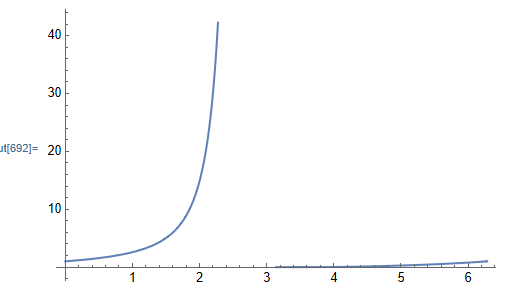Again, I know I can do the following in Maple

```plot(sol,x=0..2*Pi,view=[default,-1..20]);
```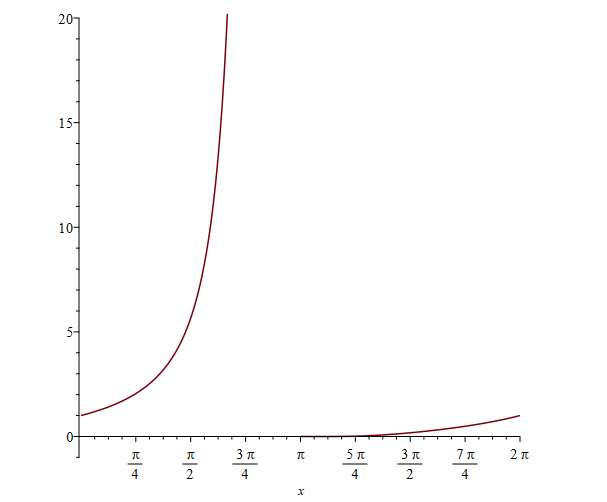The problem is that I am doing this in a program, which only gets an expression as function of x to plot, around some x location. So hard to decide what the right Y range is. It will best if Plot can determine the best view automatically.

How to use smartview to handle this? Or are there other alternative plot options for such cases?

## Error, invalid FONT specification when using axesf...

The command line print driver is still broken in Maple.  Same code works OK in the worksheet, but gives error in command line.

```restart;
p:=plot(sin(x),x=0..3,axesfont=[12,12]);
full_file_name:=cat("C:\\TMP\\tmp.ps");
plotsetup(ps,plotoutput=full_file_name);
print(p);
plotsetup(default):
```

No problem in the worksheet.  When putting the above code in file t.mpl and running it from DOS command line, it gives this error

```>"C:\Program Files\Maple 2021\bin.X86_64_WINDOWS\cmaple.exe"  t.mpl

|\^/|     Maple 2021 (X86 64 WINDOWS)
._|\|   |/|_. Copyright (c) Maplesoft, a division of Waterloo Maple Inc. 2021
<____ ____>  Waterloo Maple Inc.
|       Type ? for help.
> p:=plot(sin(x),x=0..3,axesfont=[12,12]):
> full_file_name:=cat("C:\\TMP\\tmp.ps");
> plotsetup(ps,plotoutput=full_file_name);
> print(p);

Error, invalid FONT specification

> plotsetup(default):
> quit```

The fix is to remove axesfont=[12,12] from the plot command.

Is this known bug in Maple 2021.1 on windows 10?

## Suppress Warnings...

I create and use the variable e (for elementary charge) often in my work.  I recently upgraded from version 2019 to version 2021 .  My workbooks now show the following warning when I run anything using my e vaiable.

Warning, if e is meant to be the exponential e, use command/symbol completion or palettes to enter this special symbol, or use the exp function

Is there a way to turn off this warning?  It is very annoying and a bit embarassing when I demo anything.

Thanks

## Has anyone try using Maple 2021 in Window 11? ...

Hi Maple Users,

Microsoft is enticing me to upgrade to Windows 11 from 10 for free. I am a bit hesitant because I don't know if Maple 2021 will continue working as well as in Windows 10. Does anyone have any thoughts or wisdom to share.

Thank you.